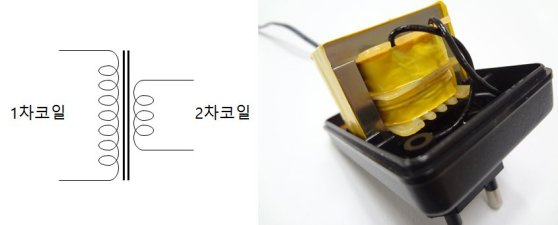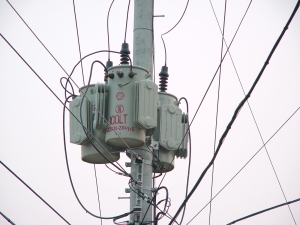# Electric Transformer

* We assume an ideal transformer where the excitation current is “0”.

A transformer is

a device for simply changing the voltage of an alternating voltage.
Transformers make it easy to change the voltage of an AC power source.
On the other hand, in the case of DC power supply, it is difficult to change the voltage like that way.

The transformer consists of a primary coil and a secondary coil.
When AC power is applied to the primary coil, a change in the magnetic field causes a secondary voltage to the secondary coil.
In general, transformers are made by putting iron cores in a coil. If you put the iron core in the coil, the magnetic field will be concentrated on the iron core and will react better to changes in the magnetic field.The above picture is a small power transformer that can be found in many household adapters. If you connect the home power, you can get the changed voltage.

Voltage change of transformer

The transformer can change the voltage freely depending on the number of turns of the primary and secondary coils.
The voltage induced in the secondary coil follows the following rule:

$V_1 : V_2 = N_1 : N_2 = \frac{1}{I_1} : \frac{1}{I_2}$

V : Voltage
N : the number of turns
I : Current

A boost transformer that boosts the voltage can seem like getting energy for free.
However, as the voltage rises, the current drops, so there is no gain in total energy. After all, electric power consumption is constant.

The following picture is a transformer suspended at a utility pole.This transformer converts high voltages into low voltages. The lowered voltage is supplied to the home.
High voltage is supplied until the utility pole. Because the current can be reduced in condition of high voltage. When the current reduced, the wire can be made thinner. And it can save the energy wasted through the wire.